Ex 1.5

Chapter 1 Class 11 Sets
Serial order wise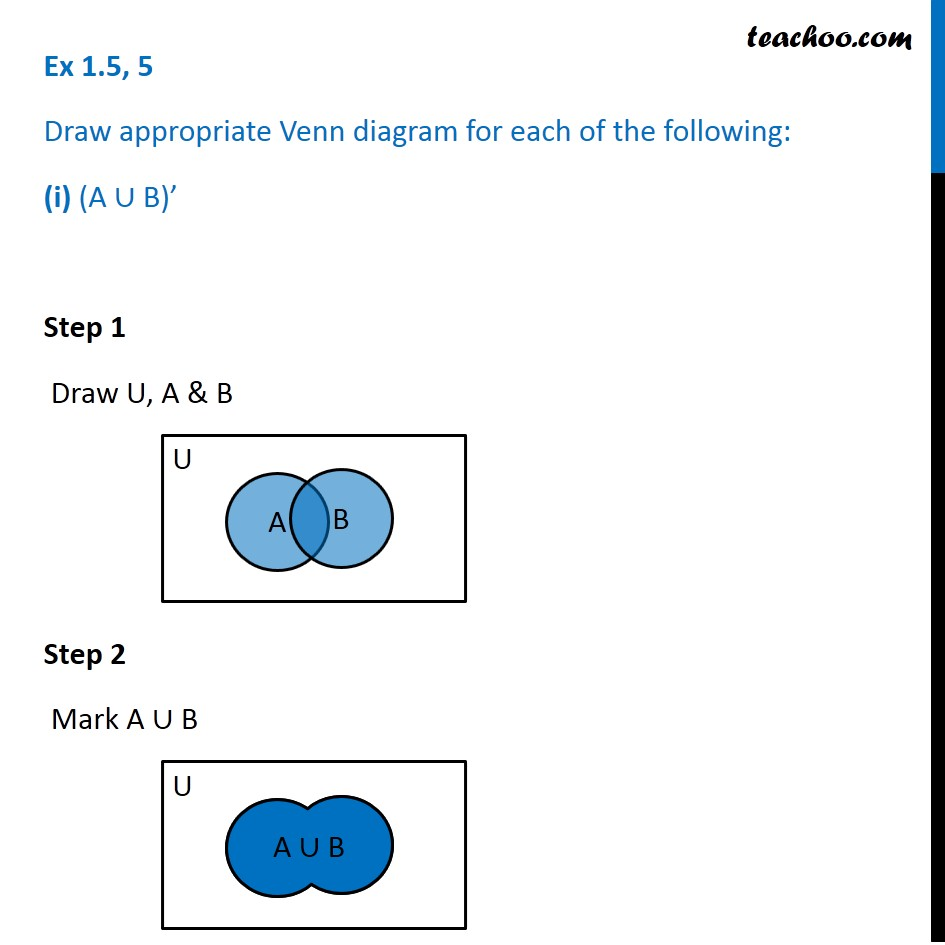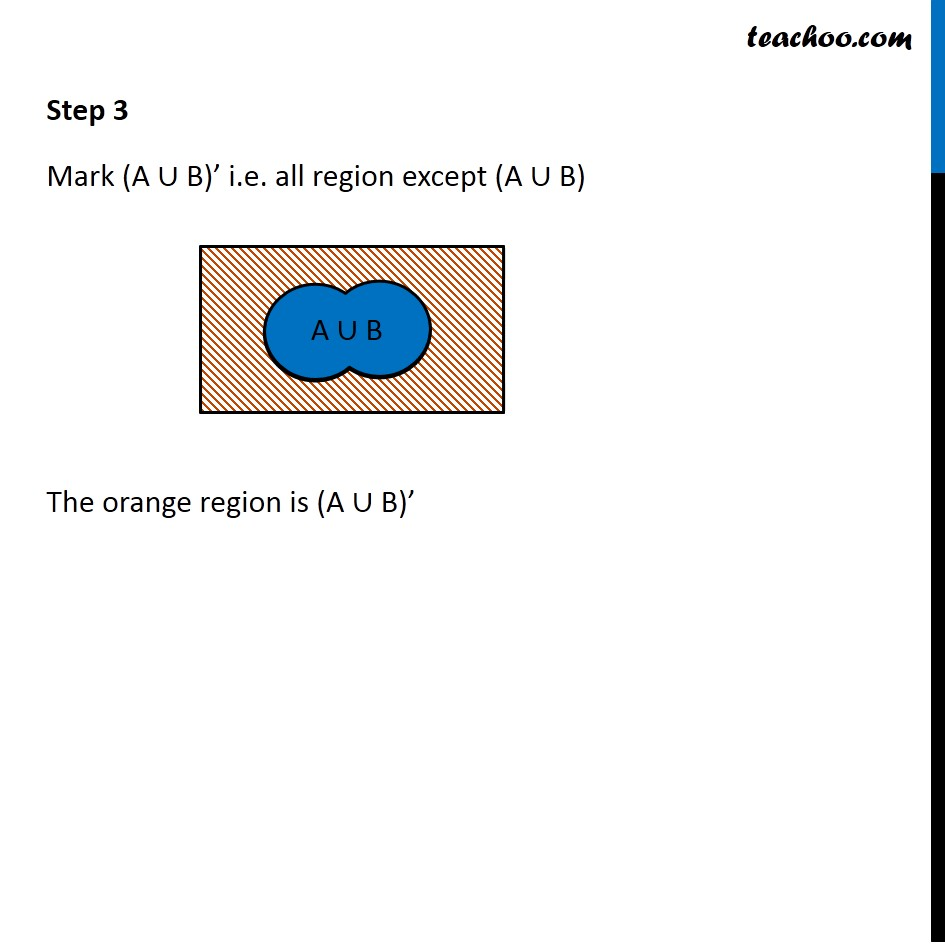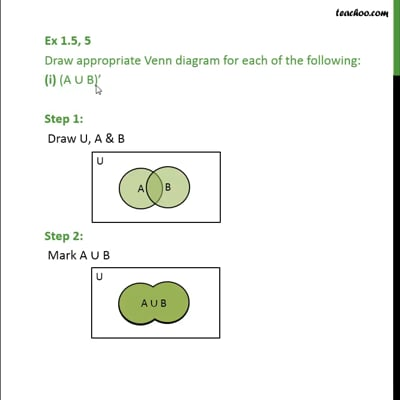This video is only available for Teachoo black users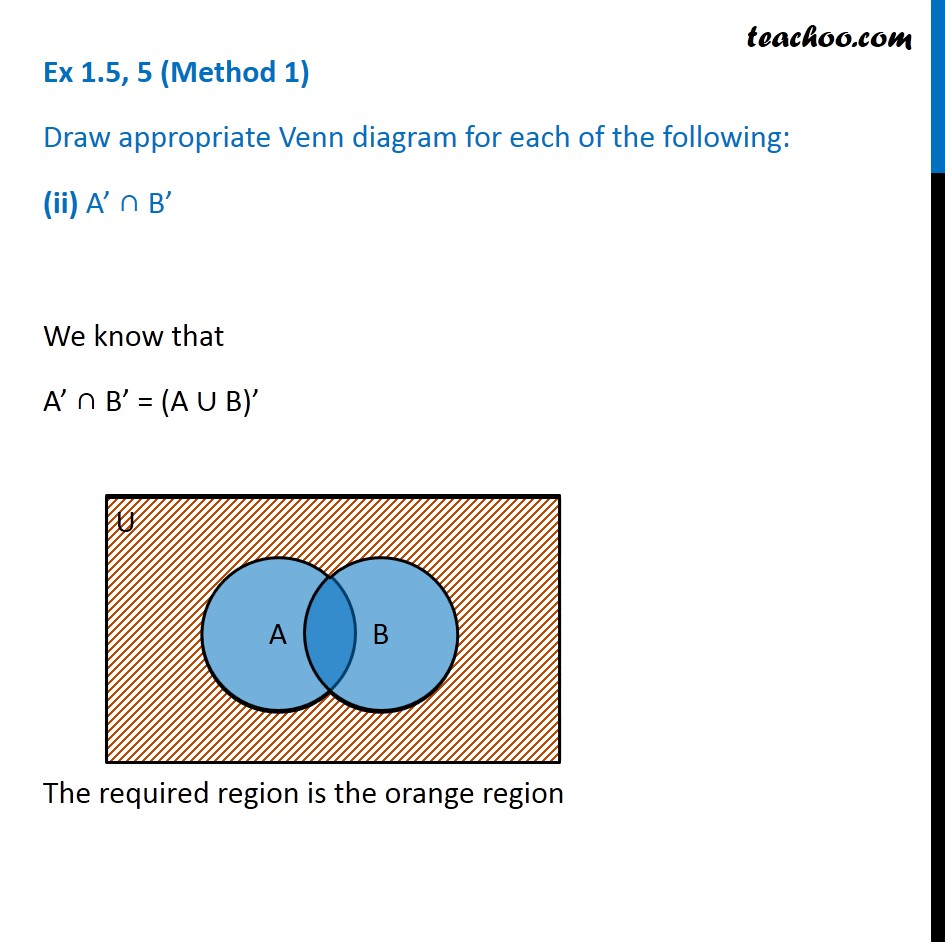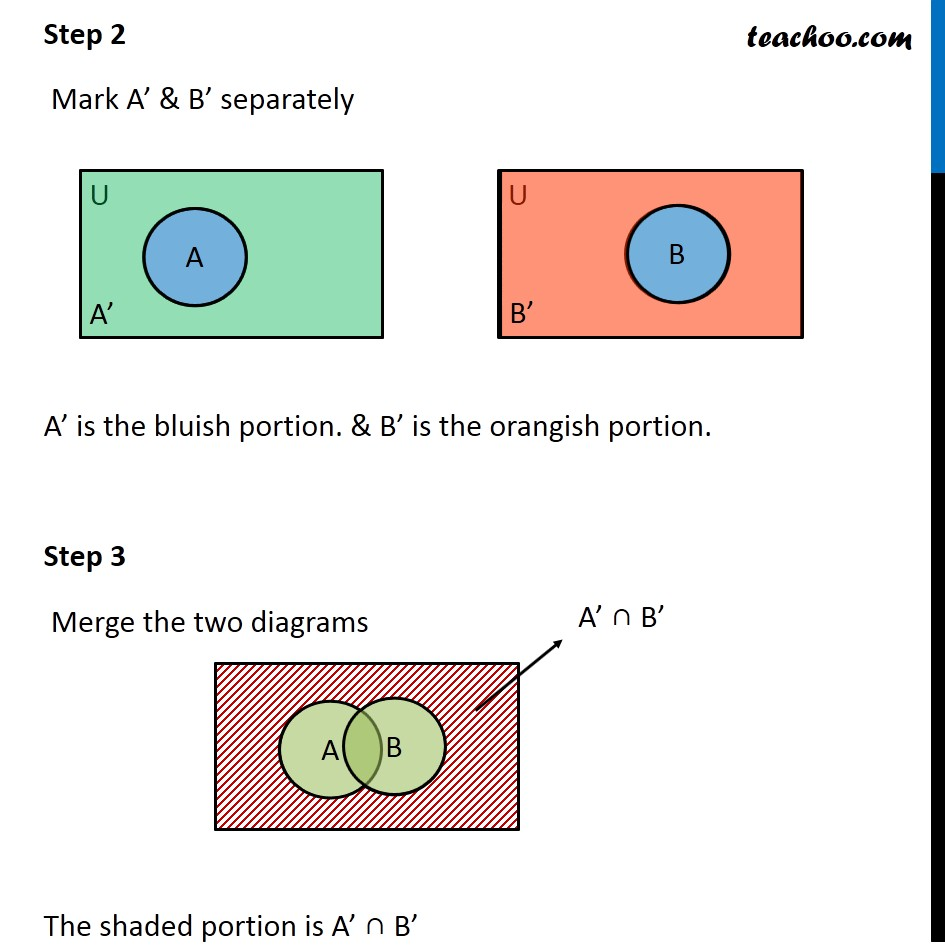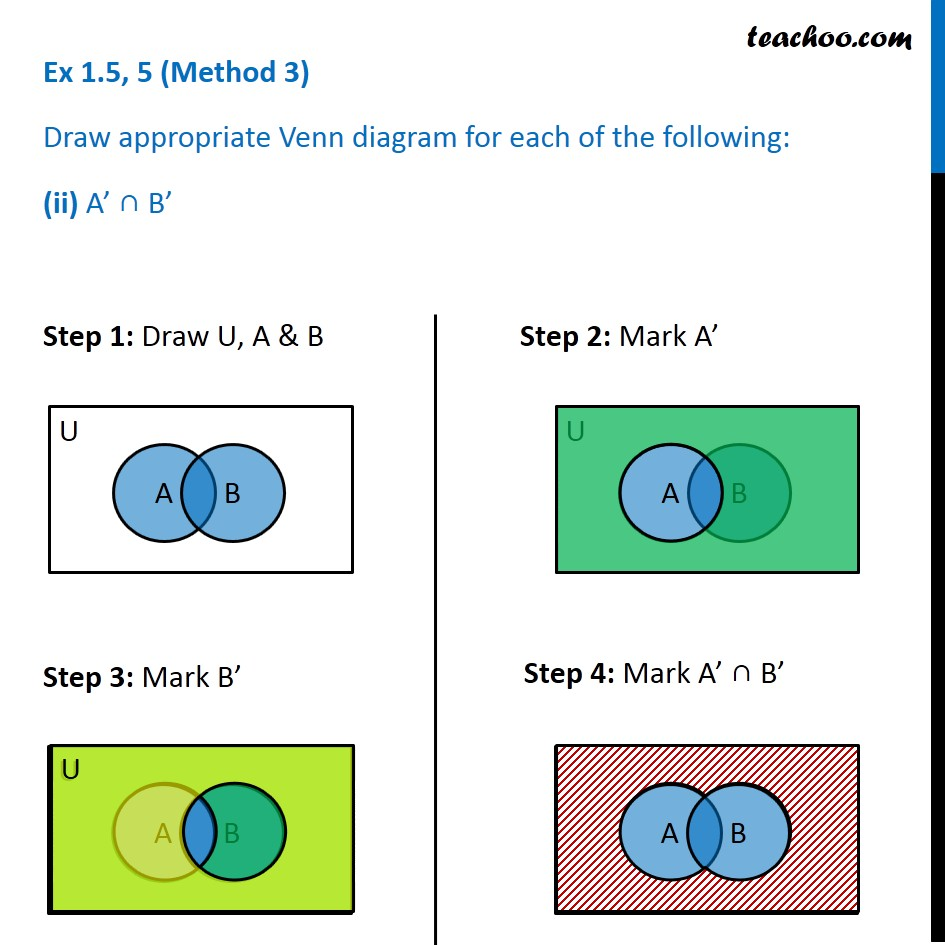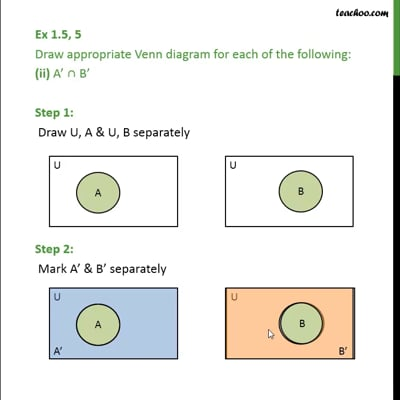This video is only available for Teachoo black users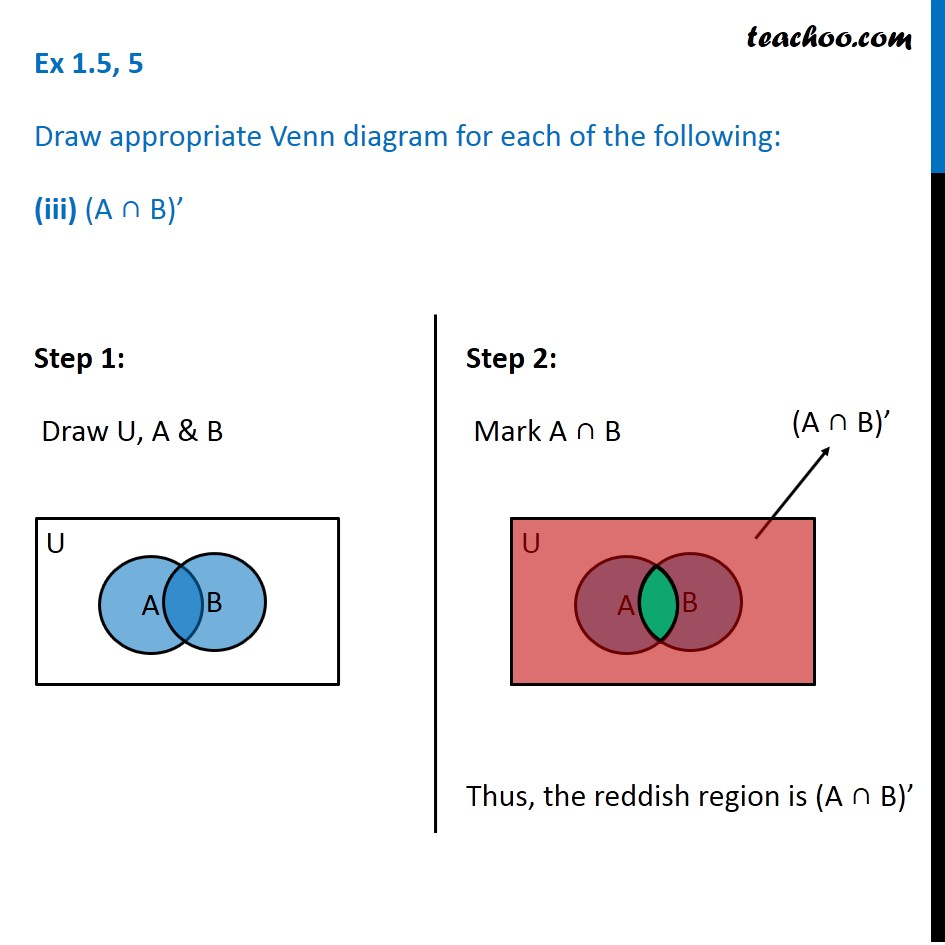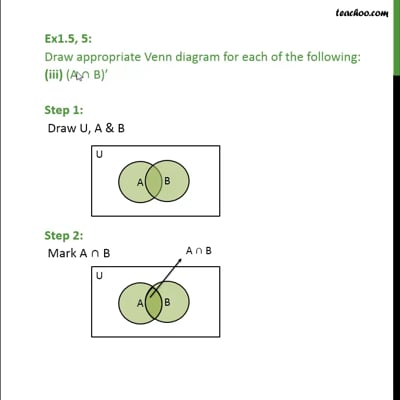This video is only available for Teachoo black users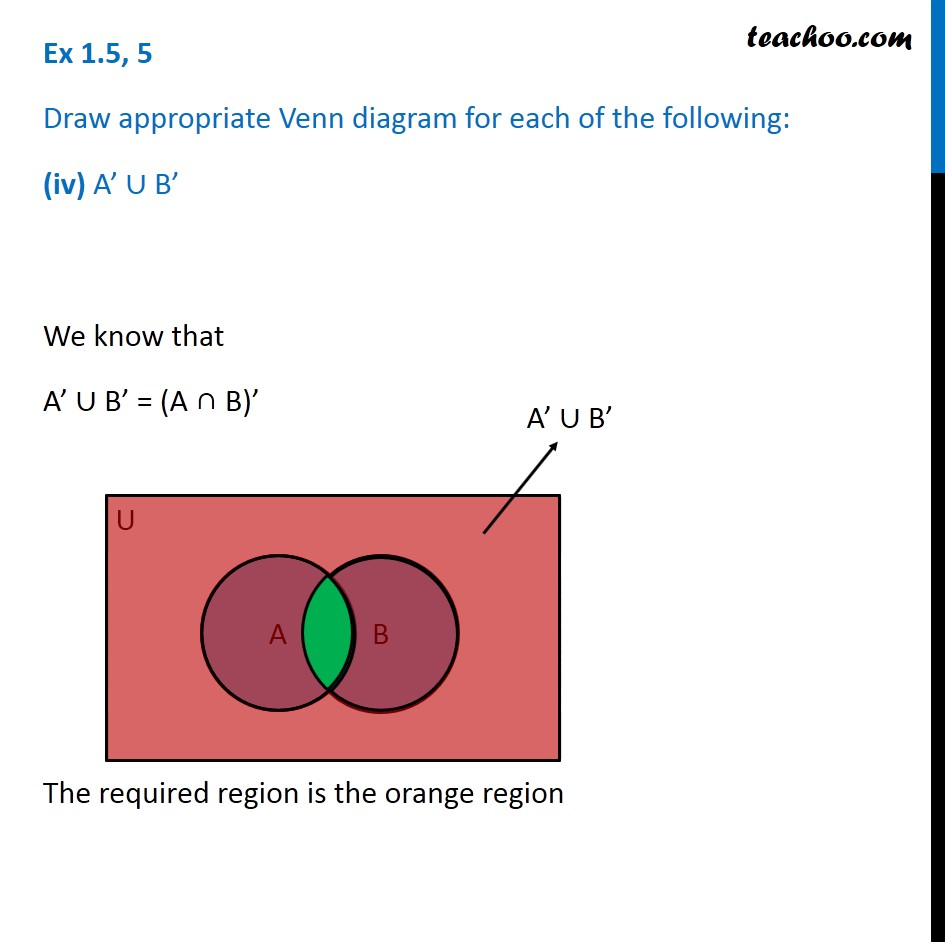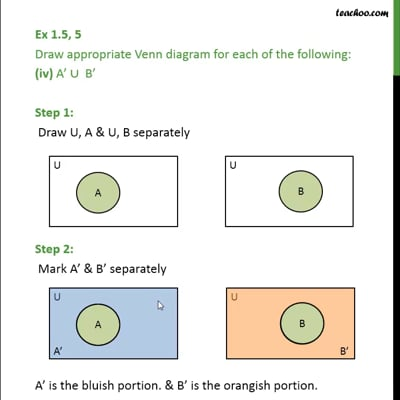This video is only available for Teachoo black users

Maths Crash Course - Live lectures + all videos + Real time Doubt solving!

### Transcript

Ex 1.5, 5 Draw appropriate Venn diagram for each of the following: (i) (A ∪ B)’ Step 1 Draw U, A & B Step 2 Mark A ∪ B Step 3 Mark (A ∪ B)’ i.e. all region except (A ∪ B) The orange region is (A ∪ B)’ Ex 1.5, 5 (Method 1) Draw appropriate Venn diagram for each of the following: (ii) A’ ∩ B’ We know that A’ ∩ B’ = (A ∪ B)’ The required region is the orange region Ex 1.5, 5 (Method 2) Draw appropriate Venn diagram for each of the following: (ii) A’ ∩ B’ Step 1 Draw U, A & U, B separately Step 2 Mark A’ & B’ separately A’ is the bluish portion. & B’ is the orangish portion. Step 3 Merge the two diagrams The shaded portion is A’ ∩ B’ Ex 1.5, 5 (Method 3) Draw appropriate Venn diagram for each of the following: (ii) A’ ∩ B’ Step 1: Draw U, A & B Step 2: Mark A’ Ex 1.5, 5 Draw appropriate Venn diagram for each of the following: (iii) (A ∩ B)’ Step 1: Draw U, A & B Step 1: Draw U, A & B Ex 1.5, 5 Draw appropriate Venn diagram for each of the following: (iv) A’ ∪ B’ We know that A’ ∪ B’ = (A ∩ B)’ The required region is the orange region